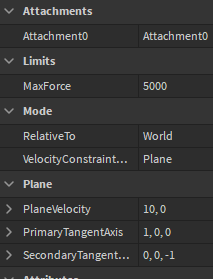# What is the backwards compatible alternative to BodyVelocity's replacement?

Greetings! I am currently switching a BodyVelocity to LinearVelocity. However, I break my game when I try switching:

The kart shouldn’t be floating.

What properties are
`BodyVelocity.Velocity`
and
`BodyVelocity.MaxForce` backwards compatible.

Here is what I tried before:

``````local BodyVelocity = Instance.new("BodyVelocity")
BodyVelocity.Velocity = Vector3.new(0, 0, 0)
BodyVelocity.MaxForce = Vector3.new(0, 0, 0)
BodyVelocity.Parent = Car.Chassis

local LinearVelocity = Instance.new("LinearVelocity")
LinearVelocity.Attachment0 = ChassisAttachment
LinearVelocity.LineDirection = Vector3.new(0, 0, 0)
LinearVelocity.PrimaryTangentAxis = Vector3.new(0, 0, 0)
LinearVelocity.Parent = Car.Chassis
``````

Or am I doing everything correct and I need to update BodyAngularVelocity as well?

Also if anyone at Roblox is reading this, can you add an article on the Developer Documentation on how to switch instead of saying what to use? That would make my life 10x easier.

If you can help, please let me know. Thanks!

2 Likes

In your use case where you want MaxForce to be 0 on the Y axis it’s possible with LinearVelocity, by setting VelocityConstraintMode to `Plane`, RelativeTo to `World` and the two axes to the X and Z axes respectively. Notice Z is (0, 0, -1) and not (0, 0, 1). It should look like this:Now you set the velocity using PlaneVelocity instead.

1 Like

Hi, I am still getting the footing kart thing. When I use the deprecated BodyVelocities instead, my kart doesn’t float.

``````--local BodyVelocity = Instance.new("BodyVelocity")
--BodyVelocity.Velocity = Vector3.new(0, 0, 0)
--BodyVelocity.MaxForce = Vector3.new(0, 0, 0)
--BodyVelocity.Parent = Car.Chassis

local LinearVelocity = Instance.new("LinearVelocity")
LinearVelocity.VelocityConstraintMode = Enum.VelocityConstraintMode.Plane
LinearVelocity.RelativeTo = Enum.ActuatorRelativeTo.World
LinearVelocity.Attachment0 = ChassisAttachment
LinearVelocity.PlaneVelocity = Vector2.new(0, 0)
LinearVelocity.PrimaryTangentAxis = Vector3.new(0, 0, 0)
LinearVelocity.Parent = Car.Chassis
``````

PrimaryTangentAxis should be (1, 0, 0) and SecondaryTangentAxis (0, 0, -1). Seems like you’ve set it to (0, 0, 0). Let me know if it still doesn’t work